## General Question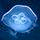# If I have a 77% as my current grade and get a 54/54 on my Chapter test what is my current percentage in my class?

Asked by Keenanduey (28) November 16th, 2015

I need help calculating my grade if I have a 77% and 54/54 on A Chapter test what is my current grade

Observing members: 0Composing members: 0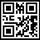Not enough information. We don’t know how the teacher calculates grades (straight line or a curve?). We also don’t know how many other tests and assignments make up the 77%.

So it’s impossible to tell how this will affect your grade without knowing more about how they are figured in the first place.

elbanditoroso (28860)“Great Answer” (2) Flag as…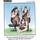89.5% if they are equally weighted.

talljasperman (21858)“Great Answer” (0) Flag as…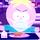Not enough information.
How many chapter tests have you had so far, and are they equally weighted?

filmfann (47842)“Great Answer” (2) Flag as…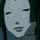Google weighted averages, also find out if there will be a curve.

ARE_you_kidding_me (19773)“Great Answer” (1) Flag as…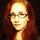Seek (34769)“Great Answer” (1) Flag as…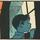You don’t tell us if the 77% is based on 154 out of 200, or if it is 1,540 out of 2,000. In the first case, 54/54 would give you a score of 208/254, which would raise your grade to 81.9%.

In the second case, it would give you a total score of 1,594 out of 2,054, which would raise your grade to 77.6%.

If this is in Math class, I suggest you ask your teacher for some help understanding how statistics work, and ho two frame a question on percentages.

zenvelo (35456)“Great Answer” (1) Flag as…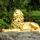I would assume there would be some weight also given to homework, attendance, no being annoying in class, etc.

Zaku (25045)“Great Answer” (1) Flag as…or